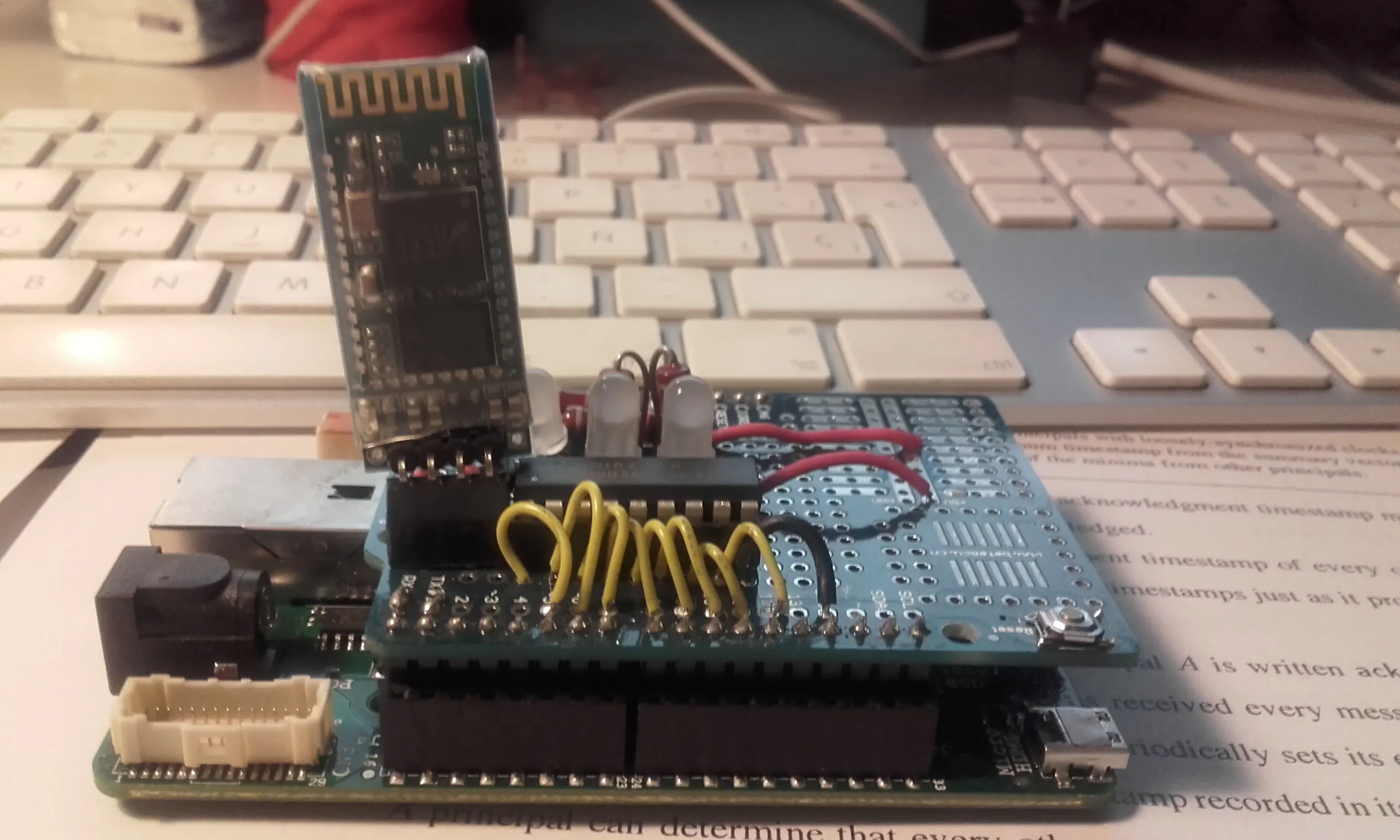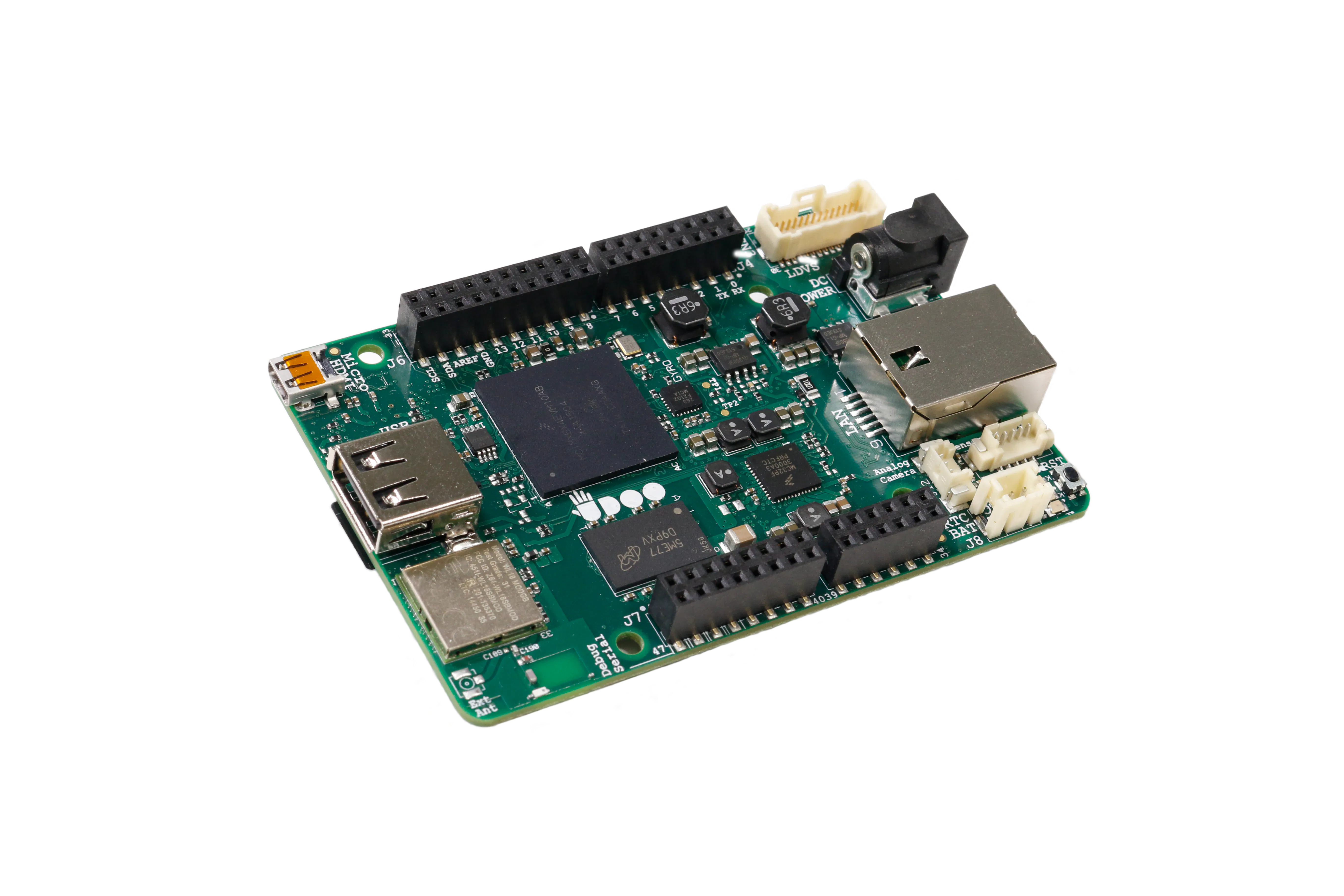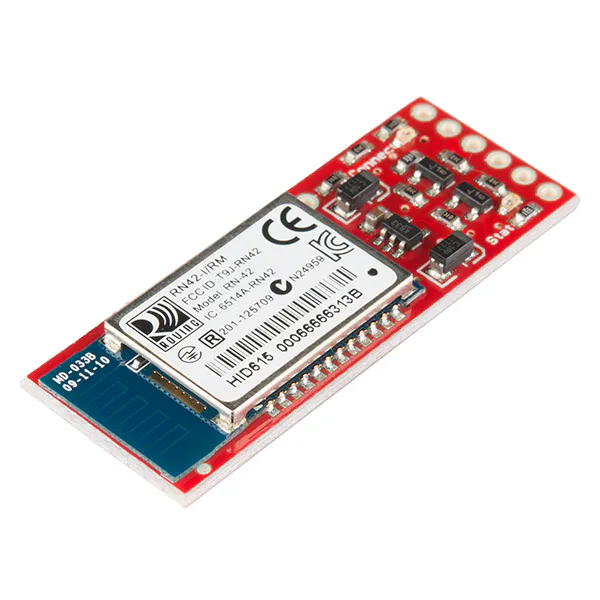# Udoo Neo External Bluetooth

Easy way to connect Neo with a mobile programming with AppInventor.

IntermediateFull instructions provided3 hours1,193## Things used in this project

### Hardware componentsUDOO NEO
×1SparkFun Bluetooth Modem - BlueSMiRF Silver
×1

## Code

### Bluetooth

Arduino
UdooNeo or arduino compatible board. We send strings wtih a mobile to a bluetooth attached to pins 0 and 1
```int ledPin[]={
5,6,7,8,9,10,11,12 };

int pines = 8;

void setup(){
Serial.begin(9600);
for (int thisPin = 0; thisPin < pines; thisPin++)  {
pinMode(ledPin[thisPin], OUTPUT);
digitalWrite(ledPin[thisPin],0);
}
}
void loop(){
if(dato == 'a'){
digitalWrite(ledPin, HIGH);
digitalWrite(ledPin, HIGH);
}
if(dato == 'b'){
digitalWrite(ledPin, LOW);
digitalWrite(ledPin, LOW);
}
if(dato == 'c')analogWrite(ledPin,255);
if(dato == 'd')analogWrite(ledPin,255);
if(dato == 'e')analogWrite(ledPin,255);
if(dato == 'f'){
for (int thisPin = 2; thisPin < 5; thisPin++){
analogWrite(ledPin[thisPin],0);
}
}
if(dato == 'g')analogWrite(ledPin,255);
if(dato == 'h')analogWrite(ledPin,255);
if(dato == 'i')analogWrite(ledPin,255);
if(dato == 'j'){
for (int thisPin = 5; thisPin < 8; thisPin++){
analogWrite(ledPin[thisPin],0);
}
}
if(dato == 'k'){
analogWrite(ledPin,0);
analogWrite(ledPin,0);
}
if(dato == 'l'){
analogWrite(ledPin,0);
analogWrite(ledPin,0);
}
if(dato == 'm'){
analogWrite(ledPin,0);
analogWrite(ledPin,0);
}
if(dato == 'n'){
for (int thisPin = 2; thisPin < 7; thisPin++){
analogWrite(ledPin[thisPin],255);
delay(200);
analogWrite(ledPin[thisPin],0);
delay(200);
}

}
delay(250);

}
```

## Credits

### Juan Carlos

8 projects • 9 followers